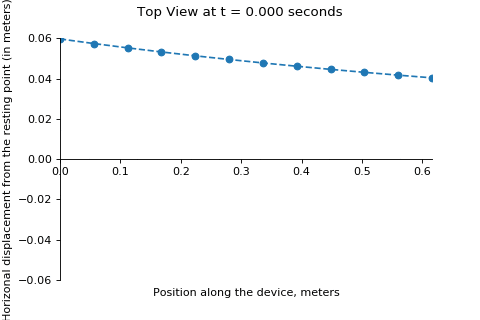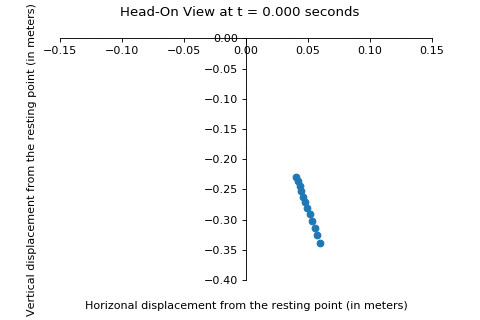# Constructing and observing a pendulum wave¶

### By Aroop "FinlayDaG33k" Roelofs¶

#### Author's Note's¶

I am no mathmatician of physicist in any way, my primary occupation is as a webdeveloper (I turn pizza into websites).
I just helped out my girlfriend so some things in here might not be correct.
I hope you enjoy reading it anyways!

If you decide to use my stuff in your own project, please mention my name and put the link to the source of this document in your sources, that'd be dope <3

#### The basis of a pendulum wave¶

The basis of a pendulum wave, is that in a certain time (the time of the whole "dance" until reset - this will be named as $\Gamma$), the pendulum on the longest length string ($l_0$) will oscillate $N$ times.
The length of each successive shorter pendulum is adjusted to a length that makes it execute one additional oscillation in this same time ($\Gamma$). $\Gamma$ will be defined here as $60 seconds$ since this number is a good, even duration after which the dance wil reset and repeat.

The dance will reset and repeat infinitely, assuming that air resistance is non-existent. But in reailty, all pendulums will come to a stop after some time due to the loss of energy due to air resistance.
60 seconds should be a short enough period of time that air resistance will have little effect on the pendulums over the period $\Gamma$.

In my small research, I have noticed that other pendulum waves have found $N = 51$ to be a good number when used in combination with $\Gamma = 60 seconds$.
Changing N would make the "dance" quicker or slower and others have found 51 oscillations over 60 seconds (for the longest pendulum) to provide a nice viewing speed, so I will use $N = 51$ as well.
Therefore, if 12 pendulums are used (which also worked well in creating other pendulum wave machines), the 1st pendulum ($l_0$) undergoes 51 oscillations in the timespan of $\Gamma$ while the 12th ($l_{11}$ - the shortest) undergoes 62 oscillations (51 oscillations + 11 pendulums from $l_0$) in this same $\Gamma = 60 seconds$ (hooray for science).

#### Some notes¶

• The derivation below assumes that the pendulum only operates under the small angle approximation where $\sin(\theta) = \theta$ (in radians).
Which basically means that the pendulum should not exceed angles of about ten degrees from it's resting position.
• The period of a pendulum can be written as $T = 2{\pi}\sqrt{\frac{l}{g}}$

#### Deriving $l$¶

Since $T = \frac{seconds}{oscillation}$, the period of the longest pendulum is $T = \frac{\Gamma}{N}$
Because other pendulums undergo $N$ + (pendulums from the first) oscillations, their period can be written as as function of pendulems from the first: $T(n) = \frac{\Gamma}{N + n}$

Knowing (read: looking up on Google) that $T = 2{\pi}\sqrt{\frac{l}{g}}$, $T(n)$ could be substituted for $T$, giving us $\frac{\Gamma}{N + n} = 2\pi \sqrt{\frac{l(n)}{g}}$

$l$ can then be solved as:

• $\frac{\Gamma}{2\pi(N + n)} = \sqrt{\frac{l(n)}{g}}$
• $(\frac{\Gamma}{2\pi(N + n)})^2 = \frac{l(n)}{g}$
• $l(n) = g(\frac{\Gamma}{2\pi(N + n)})^2$

For our pendulum wave, consisting of 12 pendulums, I have written the following Python 3 code to solve the requires $l$ values (with $n$ ranging from 0 to 11 for the longest to shortest pendulums):

In :
# Import requires libraries
import math
from math import pi

# Define some variables
lengths_meters = []  # Length in Meters, EAT THAT YOU IMPERIAL PEOPLE!
gamma = 60  # Our time in seconds
N = 51

# Where n is the index of the pendulum (starting at 0)
length = lambda n: 9.81 * (gamma/ (2*pi*(N + n)))**2

# 12 pendulums, indexed 0-11
for n in range(12):
lengths_meters.append(length(n))

# Round our lengths to 3 decimals
lengths_meters_rounded = []
for length in lengths_meters:
lengths_meters_rounded.append(round(length, 3))

# Print our lengths
print("Lengths in meters: {}".format(lengths_meters_rounded))

Lengths in meters: [0.344, 0.331, 0.318, 0.307, 0.296, 0.285, 0.275, 0.266, 0.257, 0.248, 0.24, 0.233]


From these values, the pendulum was built.
Please note that the lengths listed above are from the pivot point of the pendulums to the centers of the mass of the balls on the other side, so the actual strings will be slightly shoters than the numbers given above.
The pendulums where spaced with 1.27 centimeters between the balls, meaning that the strings were hung about 5.6 centimeters apart.

## Viewing the device from above¶

In order to demonstrate the device, I have created this visualization as it can be seen from above.
It simulates the position of the center of mass for each ball as if the pendulum was started with the balls all at 10º from their resting position.
A dashed line has been drawn to show the approximate pattern of the pendulums

In :
# Import required libraries
import numpy as np
import matplotlib.pyplot as plt
from matplotlib import animation

# Set the backend of matplotlib to the 'inline' backend
%matplotlib inline

# Set the pivot point
location = np.linspace(0, 0.056*11, 12)

# Create some functions
func_theta = lambda theta_i, n, t: theta_i * math.cos((2 * pi * t) / (60 / (51 + n)))
func_dispx = lambda l, theta: l * math.sin(theta)
func_dispy = lambda l, theta: -1 * l * math.cos(theta)

def init1():
line.set_data([], [])
return (line,)

def animate1(t):
displacementArr = []
for n in range(12):
displacementArr.append(func_dispx(lengths_meters[n], func_theta(math.radians(10), n, t)))
fig.suptitle("Top View at t = {:.3f} seconds".format(round(t, 3)), fontsize=12)
line.set_data(location, np.array(displacementArr))
return (line,)

# Set up the figure, the axis and the plot we want to animate
fig, ax = plt.subplots()
ax.set_xlim([0, location])
ax.set_ylim([-0.06, 0.06])
line, = ax.plot([], [], linestyle='--', marker='o')
plt.xlabel("Position along the device, meters")
plt.ylabel("Horizonal displacement from the resting point (in meters)")
fig.suptitle("Top View at t = 0 seconds", fontsize=12)
ax.spines['left'].set_position('zero')
ax.spines['right'].set_color('none')
ax.spines['bottom'].set_position('zero')
ax.spines['top'].set_color('none')

# Run the animation and save it as a gif with 60fps... 60 FPS MASTER RACE!
fps=60
anim = animation.FuncAnimation(fig, animate1, init_func=init1, frames=np.linspace(0, 60, 60*fps//2), blit=True)
anim.save('animation_top_bottom.gif', dpi=80, writer='imagemagick', fps=60)
plt.close()## Visualizing the device from the front¶

Below, I have made a visualization of the device as seen from the front where $l_{11}$ is the first pendulum in the view.
Again, this simulates the position of the center of mass for each ball as if the pendulum was started with the balls all at 10º from their resting position.
This time, no dotted line has been drawn.

In :
# Set up the figure, the axis, and the plot we want to animate
fig, ax = plt.subplots()
ax.set_xlim([-0.15, 0.15])
ax.set_ylim([-0.4, 0])
line, = ax.plot([], [], linestyle='', marker='o')
plt.xlabel("Horizonal displacement from the resting point (in meters)")
plt.ylabel("Vertical displacement from the resting point (in meters)")
fig.suptitle("Top View at t = 0 seconds", fontsize=12)
ax.spines['left'].set_position('zero')
ax.spines['right'].set_color('none')
ax.spines['bottom'].set_position('zero')
ax.spines['top'].set_color('none')

# Create some functions
def init2():
line.set_data([], [])
return (line,)

def animate2(t):
x_pos = []
y_pos = []
for n in range(12):
x_pos.append(func_dispx(lengths_meters[n], func_theta(math.radians(10), n, t)))
y_pos.append(func_dispy(lengths_meters[n], func_theta(math.radians(10), n, t)))
fig.suptitle("Head-On View at t = {:.3f} seconds".format(round(t, 3)), fontsize=12)
line.set_data(np.array(x_pos), np.array(y_pos))
return (line,)

# Run the animation and save it as a gif with 60fps... 60 FPS MASTER RACE!
fps = 60
anim = animation.FuncAnimation(fig, animate2, init_func=init2, frames=np.linspace(0, 60, 60*fps//2), blit=True)
anim.save('animation_front_rear.gif', dpi=80, writer='imagemagick', fps=60)
plt.close()## Some Observations¶

• Compared to a real-life machine, the visualizations are more accurate because, as I've already mentioned, the air resistance and friction would have a major impact over the 60s $\Gamma$ of the real-life machine, while both of these sources do not exist in the simulations seen above.
• As expected, each ball was in the same place at $t = 0s$ and $t = \Gamma = 60s$.
• Various patterns and shapes appear throughout the similation (and real experiment).
They occurred due to the pendulums their positions getting out of sinc due to their different lengths and therefore, different oscillation times.
The pendulums started in sync, but the constant difference of 1 oscillation over 60 seconds slowly put them out of sync with their neighbouring pendulums.
• Making animations with Matplotlib is a major pain in the buttocks.
• Looking at the animations for too long can make you feel like you're having a trip.